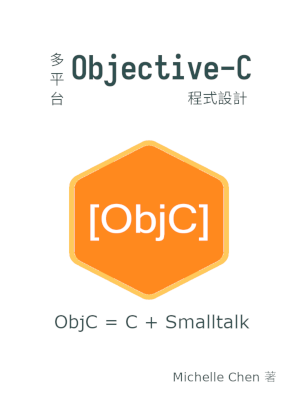#用 Objective-C++ 混合 C++ 和 Objective-C 程式碼

## 前言

Objective-C++ 並不是新的語言，而是容許 Objective-C 和 C++ 程式碼混合撰寫的模式。由於 Objective-C 的部分和原本的語法相同，我們只要加上和 C++ 相關的部分即可。本文會以兩個簡短範例來說明如何撰寫 Objective-C++ 程式。

## 撰寫第一隻 Objective-C++ 程式

``````/* hello.mm */
#import <Foundation/Foundation.h>
#include <iostream>

using std::cout;
using std::endl;

int main(void)
{
NSAutoreleasePool *pool = \
[[NSAutoreleasePool alloc] init];
if (!pool)
return 1;

cout << "Hello C++" << endl;

NSLog(@"Hello Objective-C\n");

[pool drain];

return 0;
}
``````

## 編譯第一隻 Objective-C++ 程式

``````\$ clang++ -o hello hello.mm -lobjc -framework Foundation
``````

``````\$ g++ -o hello hello.mm -lobjc -lgnustep-base -I /usr/include/GNUstep -L /usr/lib/GNUstep -fconstant-string-class=NSConstantString
``````

``````\$ clang++ -o hello hello.mm -lobjc -lgnustep-base -I /usr/include/GNUstep -L /usr/lib/GNUstep -I /usr/lib/gcc/x86_64-linux-gnu/7.4.0/include -fconstant-string-class=NSConstantString
``````

## 用 Objective-C++ 包裝 C++ 類別

Objective-C++ 的主要意義不在於 Objective-C 和 C++ 的混合編碼，而是橋接 C++ 類別。因為我們在專案中混入 Objective-C++ 程式碼後，整個專案被迫要以 C++ 來寫。一般來說，我們會希望主程式仍保持純 Objective-C 的模式，只在類別中混入 Objective-C++ 的程式碼。

``````/* point.hpp */
#pragma once

class Point
{
public:
Point(double x, double y);
double x();
double y();
static double distance(Point *p, Point *q);
private:
double _x;
double _y;
};
``````

`Point` 類別的宣告相當簡單，不多做說明。

``````/* point.cpp */
#include <cmath>
#include "point.hpp"

Point::Point(double x, double y)
{
this->_x = x;
this->_y = y;
}

double Point::x()
{
return this->_x;
}

double Point::y()
{
return this->_y;
}

double Point::distance(Point *p, Point *q)
{
double dx = p->x() - q->x();
double dy = p->y() - q->y();

return sqrt(pow(dx, 2) + pow(dy, 2));
}
``````

``````/* objpoint.h */
#pragma once

#import <Foundation/Foundation.h>

#ifdef __cplusplus
extern "C" {
#endif

@interface ObjPoint : NSObject {
void *p;
}

-(ObjPoint *) init;
-(ObjPoint *) initWithX: (double) x andY: (double) y;

-(void) dealloc;

+(double) distanceBetween: (ObjPoint *) p andPoint: (ObjPoint *) q;

-(double) x;
-(double) y;
@end

#ifdef __cplusplus
}
#endif
``````

``````/* objpoint.mm */
#include <cmath>
#include "point.hpp"
#import "objpoint.h"

@implementation ObjPoint
-(ObjPoint *) init
{
return [self initWithX: 0.0 andY: 0.0];
}

-(ObjPoint *) initWithX: (double)x andY: (double)y
{
if (!self)
return self;

self = [super init];

p = (void *) new Point(x, y);
if (!p) {
[super release];
self = nil;
return self;
}

return self;
}

-(void) dealloc
{
if (!self)
return;

delete (Point *) p;
[super dealloc];
}

+(double) distanceBetween: (ObjPoint *)p andPoint: (ObjPoint *)q
{
return Point::distance((Point *) (p->p), (Point *) (q->p));
}

-(double) x
{
return ((Point *) p)->x();
}

-(double) y
{
return ((Point *) p)->y();
}
@end
``````

`ObjPoint` 的內部實作中，真正的運算並非由 `ObjPoint` 類別來負責，而是由 `Point` 類別來負責。`ObjPoint` 類別只是用來包住型別為 `Point *` 的物件 `p`，並提供適用於 Objective-C 的公開界面。

``````/* main.mm */
#import <Foundation/Foundation.h>
#include <iostream>
#import "objpoint.h"

using std::cerr;
using std::endl;

int main(void)
{
NSAutoreleasePool *pool = [[NSAutoreleasePool alloc] init];
if (!pool)
return 1;

ObjPoint *p;
ObjPoint *q;

p = [[[ObjPoint alloc] init] autorelease];
if (!p) {
cerr << "Failed to allocate p" << endl;
goto ERROR;
}

q = [[[ObjPoint alloc] initWithX: 3.0 andY: 4.0] autorelease];
if (!q) {
cerr << "Failed to allocate q" << endl;
goto ERROR;
}

if (!(5.0 == [ObjPoint distanceBetween: p andPoint: q])) {
cerr << "Wrong distance" << endl;
goto ERROR;
}

[pool drain];

return 0;

ERROR:
[pool drain];

return 1;
}
``````

``````/* main.m */
#import <Foundation/Foundation.h>
#include <stdio.h>
#import "objpoint.h"

int main(void)
{
NSAutoreleasePool *pool = [[NSAutoreleasePool alloc] init];
if (!pool)
return 1;

ObjPoint *p;
ObjPoint *q;

p = [[[ObjPoint alloc] init] autorelease];
if (!p) {
fprintf(stderr, "Failed to allocate p\n");
goto ERROR;
}

q = [[[ObjPoint alloc] initWithX: 3.0 andY: 4.0] autorelease];
if (!q) {
fprintf(stderr, "Failed to allocate q\n");
goto ERROR;
}

if (!(5.0 == [ObjPoint distanceBetween: p andPoint: q])) {
fprintf(stderr, "Wrong distance\n");
goto ERROR;
}

[pool drain];

return 0;

ERROR:
[pool drain];

return 1;
}
``````

## 使用 GCC 編譯 `Point` 程式

point.cpp 是 C++ 原始碼，使用一般的指令來編譯即可：

``````\$ g++ -c -o point.o point.cpp
``````

objpoint.mm 是 Objective-C++ 原始碼，所以要加上必要的參數：

``````\$ g++ -c -o objpoint.o objpoint.mm -I /usr/include/GNUstep -fconstant-string-class=NSConstantString
``````

``````\$ g++ -c -o main.o main.mm -I /usr/include/GNUstep -fconstant-string-class=NSConstantString
``````

``````\$ g++ -o point point.o objpoint.o main.o -lobjc -lgnustep-base -I /usr/include/GNUstep -L /usr/lib/GNUstep -fconstant-string-class=NSConstantString
``````

``````\$ gcc -c -o main.o main.m -I /usr/include/GNUstep -fconstant-string-class=NSConstantString
``````

``````\$ gcc -o point point.o objpoint.o main.o -lstdc++ -lm -lobjc -lgnustep-base -I /usr/include/GNUstep -L /usr/lib/GNUstep -fconstant-string-class=NSConstantString
``````

## 使用 Clang 編譯 `Point` 程式

``````\$ clang++ -o point point.o objpoint.o main.o -lobjc -lgnustep-base -I /usr/include/GNUstep -L /usr/lib/GNUstep -I /usr/lib/gcc/x86_64-linux-gnu/7.4.0/include -fconstant-string-class=NSConstantString
``````

## 評語

Objective-C++ 其實有很多本文未提及的限制，像是 Objective-C 的類別和 C++ 的類別不能互相繼承，Objective-C 的類別不能用命名空間等。C++ 沒有辦法解放 Objective-C，反而是 Objective-C 限制了某些 C++ 的特性。

## 電子書籍